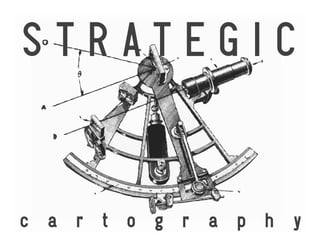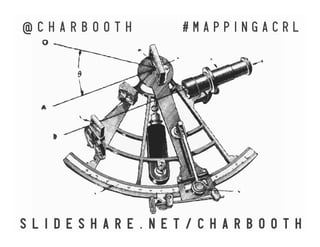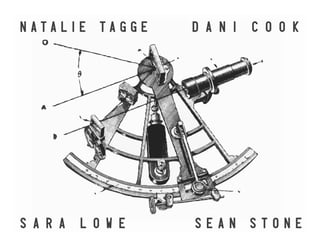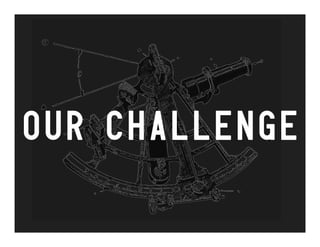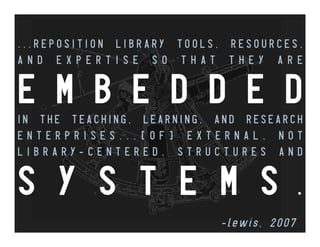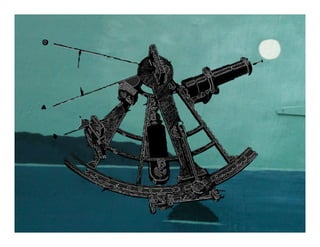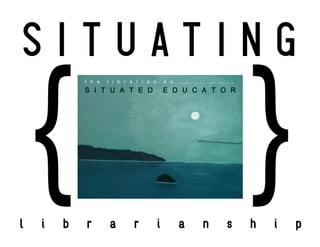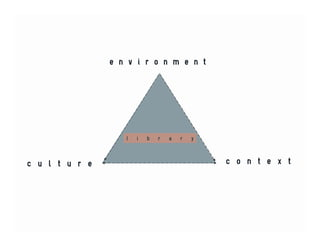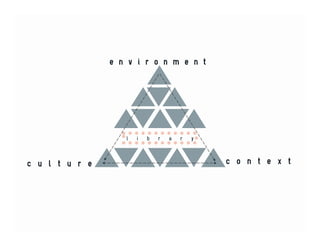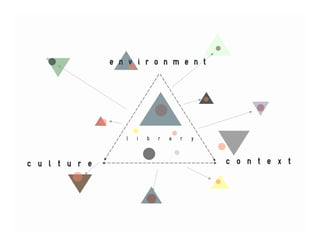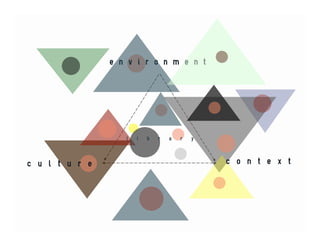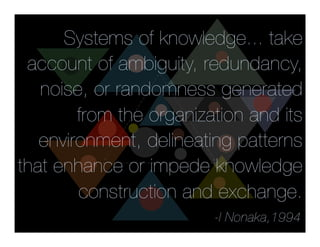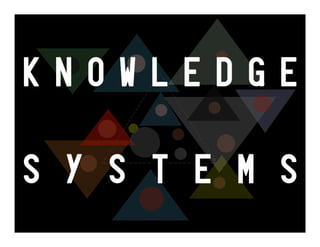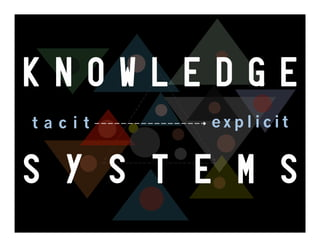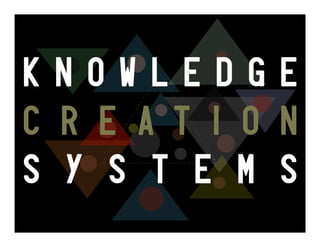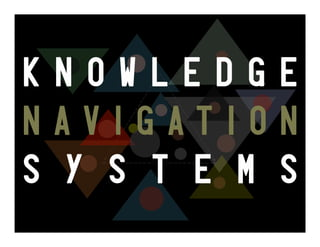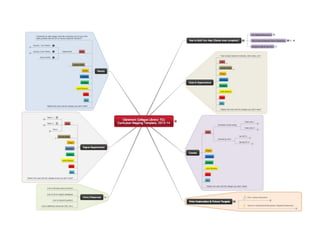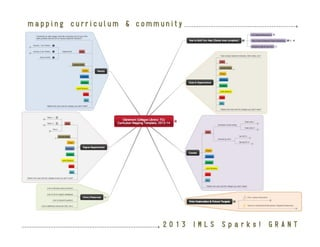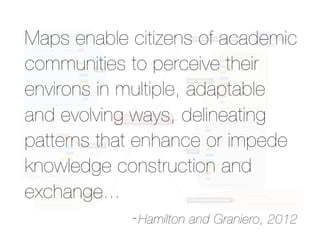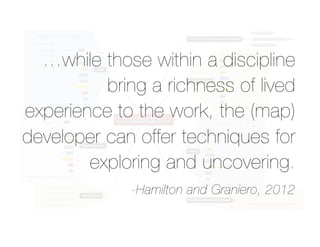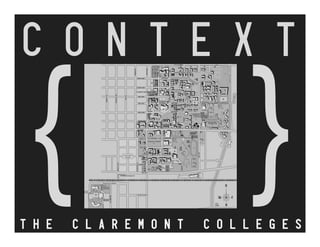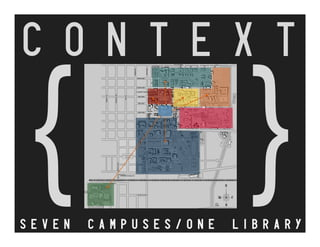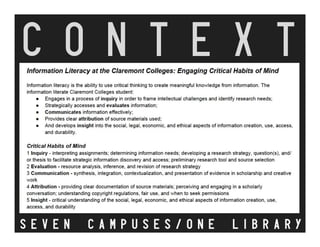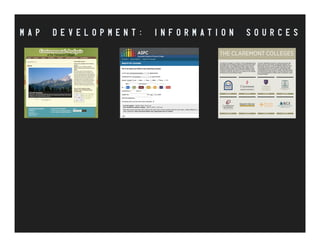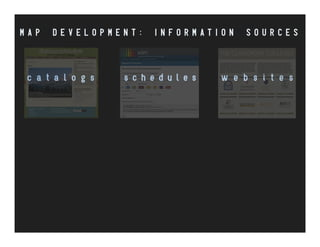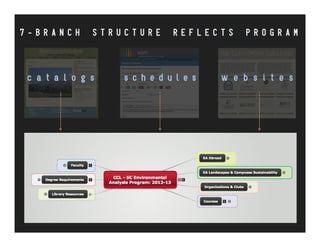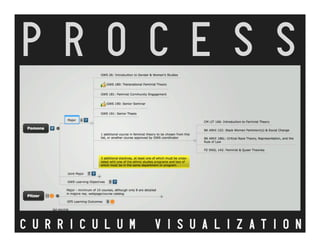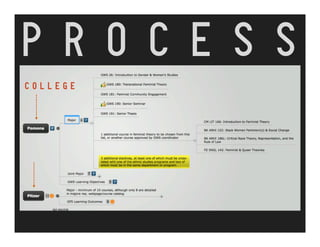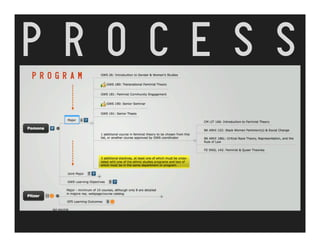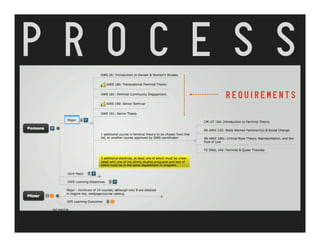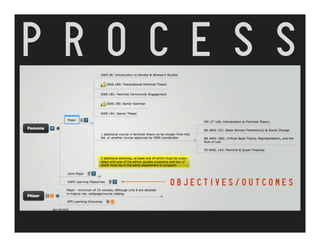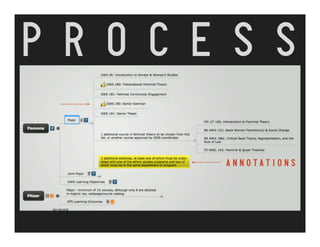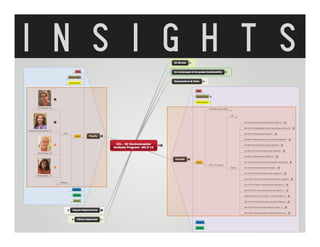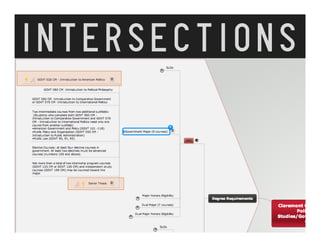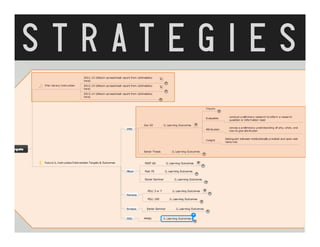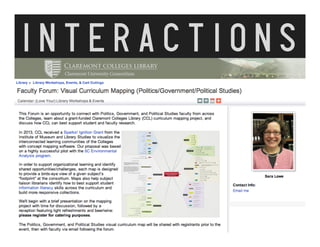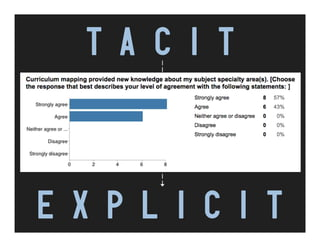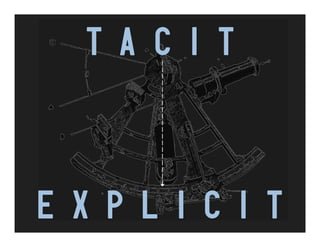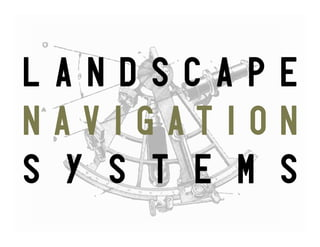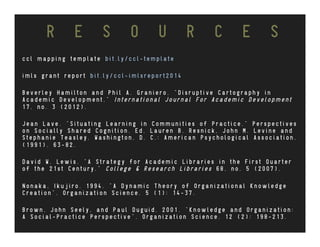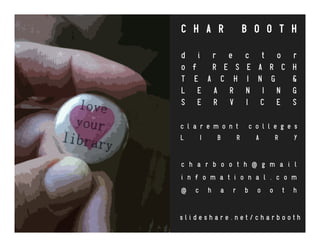1 of 41

### Strategic Cartography: Identifying IL Intersections Across the Curriculum

1. c a r t o g r a p h y S T R A T E G I C
2. S L I D E S H A R E . N E T / C H A R B O O T H @ C H A R B O O T H # M A P P I N G A C R L
3. N A T A L I E T A G G E D A N I C O O K S A R A L O W E S E A N S T O N E
4. OUR CHALLENGE
5. -lewis, 2007 . . . R E P O S I T I O N L I B R A R Y T O O L S , R E S O U R C E S , A N D E X P E R T I S E S O T H A T T H E Y A R E E M B E D D E D I N T H E T E A C H I N G , L E A R N I N G , A N D R E S E A R C H E N T E R P R I S E S . . . [ O F ] E X T E R N A L , N O T L I B R A R Y - C E N T E R E D , S T R U C T U R E S A N D S Y S T E M S .
6. l i b r a r i a n s h i p S I T U A T I N G { }
7. c u l t u r e c o n t e x t e n v i r o n m e n t l i b r a r y
8. c u l t u r e c o n t e x t l i b r a r y e n v i r o n m e n t
9. c u l t u r e c o n t e x t e n v i r o n m e n t l i b r a r y
10. e n v i r o n m e n t c u l t u r e c o n t e x t l i b r a r y
11. Systems of knowledge... take account of ambiguity, redundancy, noise, or randomness generated from the organization and its environment, delineating patterns that enhance or impede knowledge construction and exchange. -I Nonaka,1994
12. K N O W L E D G E S Y S T E M S
13. K N O W L E D G E S Y S T E M S t a c i t e x p l i c i t
14. K N O W L E D G E C R E A T I O N S Y S T E M S
15. K N O W L E D G E N A V I G A T I O N S Y S T E M S
16. m a p p i n g c u r r i c u l u m & c o m m u n i t y 2 0 1 3 I M L S S p a r k s ! G R A N T
17. Maps enable citizens of academic communities to perceive their environs in multiple, adaptable and evolving ways, delineating patterns that enhance or impede knowledge construction and exchange... -Hamilton and Graniero, 2012
18. …while those within a discipline bring a richness of lived experience to the work, the (map) developer can offer techniques for exploring and uncovering. -Hamilton and Graniero, 2012
19. { } C O N T E X T T H E C L A R E M O N T C O L L E G E S
20. { } C O N T E X T S E V E N C A M P U S E S / O N E L I B R A R Y
21. { } C O N T E X T S E V E N C A M P U S E S / O N E L I B R A R Y
22. M A P D E V E L O P M E N T : I N F O R M A T I O N S O U R C E S
23. M A P D E V E L O P M E N T : I N F O R M A T I O N S O U R C E S c a t a l o g s s c h e d u l e s w e b s i t e s
24. c a t a l o g s s c h e d u l e s w e b s i t e s 7 - B R A N C H S T R U C T U R E R E F L E C T S P R O G R A M
25. C U R R I C U L U M V I S U A L I Z A T I O N P R O C E S S
26. C O L L E G E P R O C E S S
27. P R O G R A M P R O C E S S
28. R E Q U I R E M E N T S P R O C E S S
29. O B J E C T I V E S / O U T C O M E S P R O C E S S
30. A N N O T A T I O N S P R O C E S S
31. I N S I G H T S
32. INTERSECTIONS
33. S T R A T E G I E S
34. I N T E R A C T I O N S
35. T A C I T E X P L I C I T
36. T A C I T E X P L I C I T
37. L A N D S C A P E N A V I G A T I O N S Y S T E M S
38. R E S O U R C E S c c l m a p p i n g t e m p l a t e b i t . l y / c c l - t e m p l a t e i m l s g r a n t r e p o r t b i t . l y / c c l - i m l s r e p o r t 2 0 1 4 B e v e r l e y H a m i l t o n a n d P h i l A . G r a n i e r o . " D i s r u p t i v e C a r t o g r a p h y i n A c a d e m i c D e v e l o p m e n t , " I n t e r n a t i o n a l J o u r n a l F o r A c a d e m i c D e v e l o p m e n t 1 7 , n o . 3 ( 2 0 1 2 ) . J e a n L a v e . " S i t u a t i n g L e a r n i n g i n C o m m u n i t i e s o f P r a c t i c e . " P e r s p e c t i v e s o n S o c i a l l y S h a r e d C o g n i t i o n . E d . L a u r e n B . R e s n i c k , J o h n M . L e v i n e a n d S t e p h a n i e T e a s l e y . W a s h i n g t o n , D . C . : A m e r i c a n P s y c h o l o g i c a l A s s o c i a t i o n , ( 1 9 9 1 ) . 6 3 - 8 2 . D a v i d W . L e w i s . " A S t r a t e g y f o r A c a d e m i c L i b r a r i e s i n t h e F i r s t Q u a r t e r o f t h e 2 1 s t C e n t u r y . " C o l l e g e & R e s e a r c h L i b r a r i e s 6 8 , n o . 5 ( 2 0 0 7 ) . N o n a k a , I k u j i r o . 1 9 9 4 . " A D y n a m i c T h e o r y o f O r g a n i z a t i o n a l K n o w l e d g e C r e a t i o n " . O r g a n i z a t i o n S c i e n c e . 5 ( 1 ) : 1 4 - 3 7 . B r o w n , J o h n S e e l y , a n d P a u l D u g u i d . 2 0 0 1 . " K n o w l e d g e a n d O r g a n i z a t i o n : A S o c i a l - P r a c t i c e P e r s p e c t i v e " . O r g a n i z a t i o n S c i e n c e . 1 2 ( 2 ) : 1 9 8 - 2 1 3 .
39. c h a r b o o t h @ g m a i l i n f o m a t i o n a l . c o m @ c h a r b o o t h C H A R B O O T H d i r e c t o r o f R E S E A R C H T E A C H I N G & L E A R N I N G S E R V I C E S c l a r e m o n t c o l l e g e s L I B R A R Y s l i d e s h a r e . n e t / c h a r b o o t h